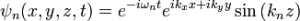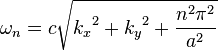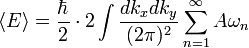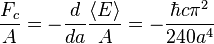## Calculation of the Casimir effect

The intensity of the force of the cashmere effect drops rapidly with distance, and it is only measurable when the distance between the objects is extremely small, on a scale below the micrometer.

In the original calculation, The free space between a pair of conductive plates was considered a distance. In this case, standing waves are easy to calculate, since the transverse component of the electric field and the normal component of the magnetic field must vanish at the surface of a conductor.Whereis the electrical component of the electromagnetic field,andare the components of the wave vector in directions parallel to the plates, andis the wave vector perpendicular to them.

The frequency for this wave would be:And the sum over the possible modes of arousal:Beingthe area of ​​the metal plates, and introducing a factor 2 due to the two possible polarizations of the wave.

And the Casimir force per unit area for ideal plates with a vacuum between them, I would be:Whereis the reduced Planck constant andthe distance between the two plates.

The Casimir effect is seen in some structures or time machines.